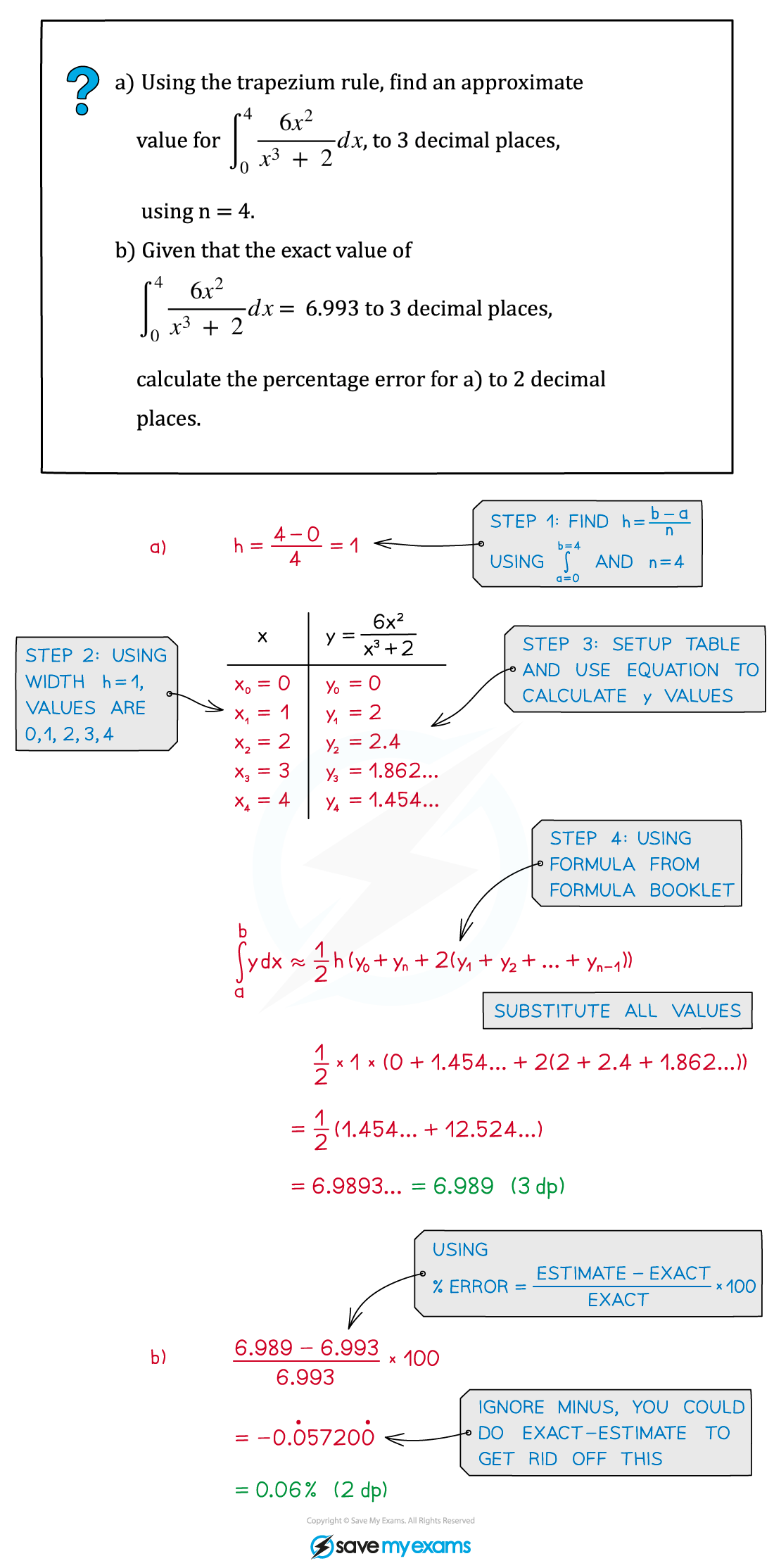# AQA A Level Maths: Pure复习笔记10.1.5 Trapezium Rule (Numerical Integration)

### Trapezium Rule (Numerical Integration)

#### What is the trapezium rule?

• The trapezium rule is a numerical method of integration used to find the approximate area under a curve
• It can be used when analytical methods of integration won’t work
• It finds an approximation of the area by finding the sum of the areas of trapeziums beneath the curve
• The formula for an estimate of the area when splitting it into n strips (trapezium) is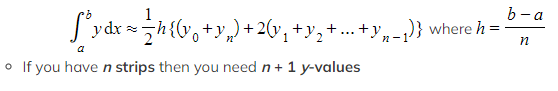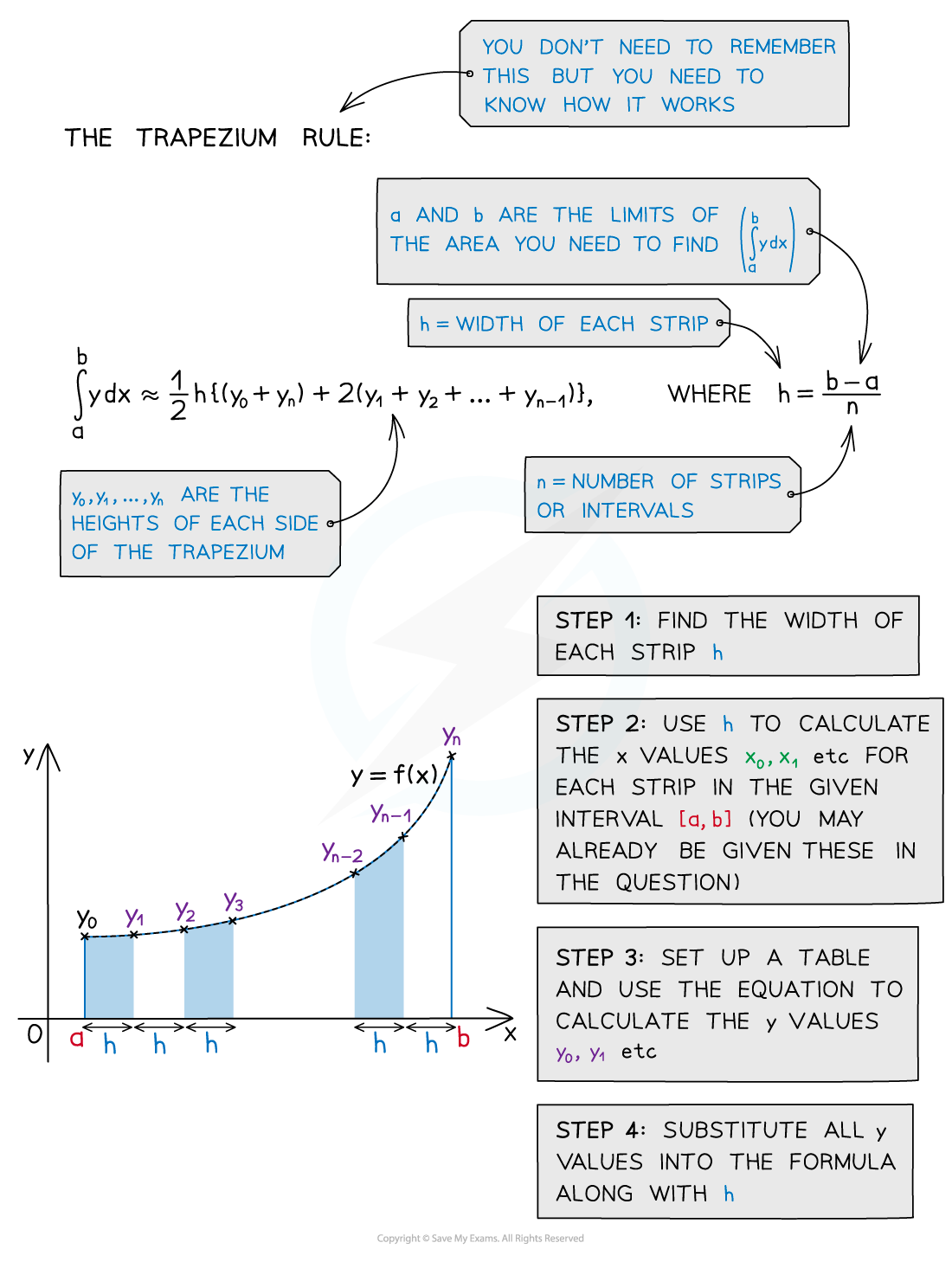#### Underestimate or overestimate?

• Depending on the shape of the curve, the trapezium rule could be an underestimate or overestimate
• The more trapezium strips used the more accurate the estimate
• Calculating the percentage error can tell you how accurate your estimate is
• Using rectangular strips can find the upper and lower bounds of the interval for the trapezium rule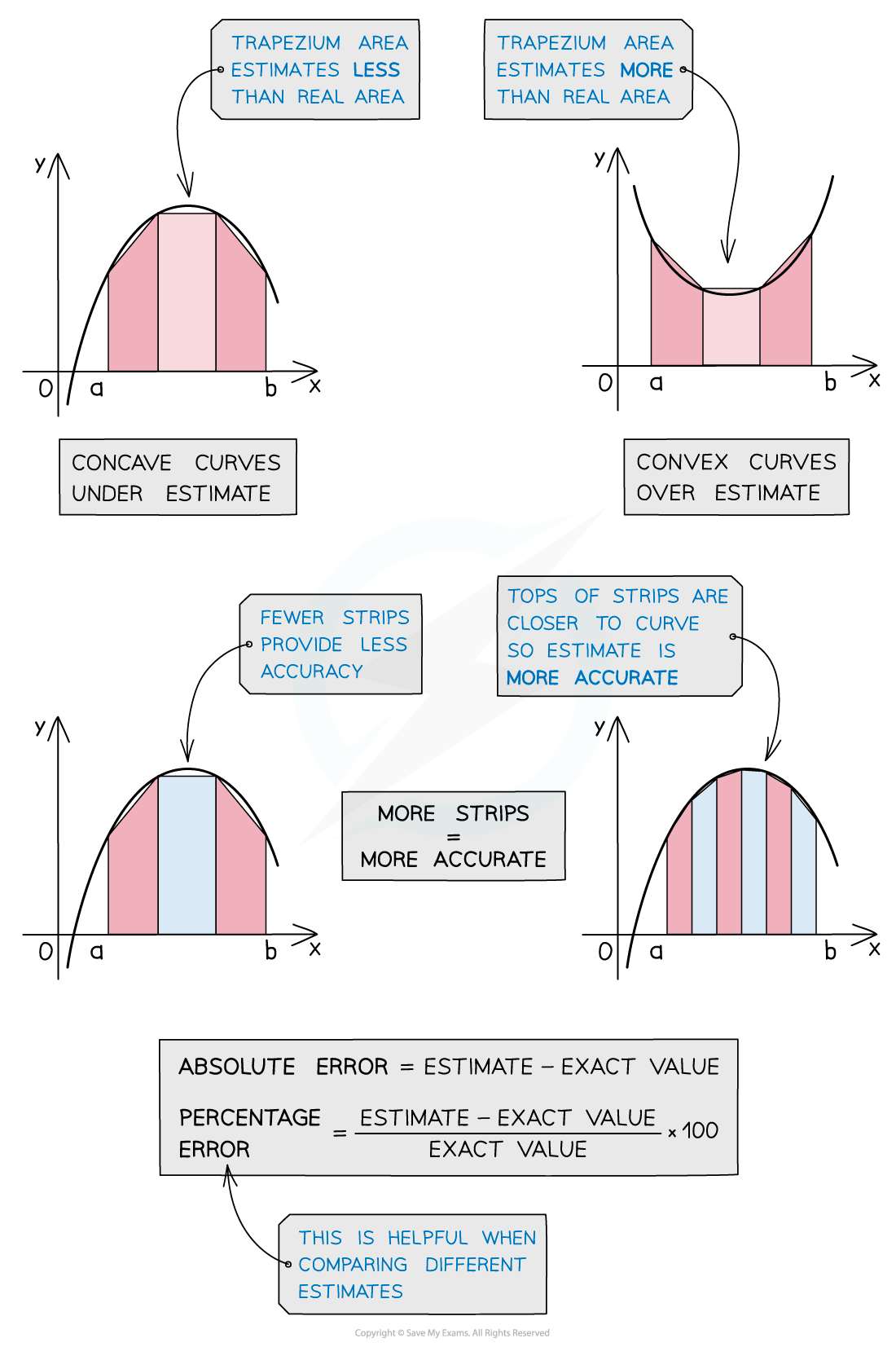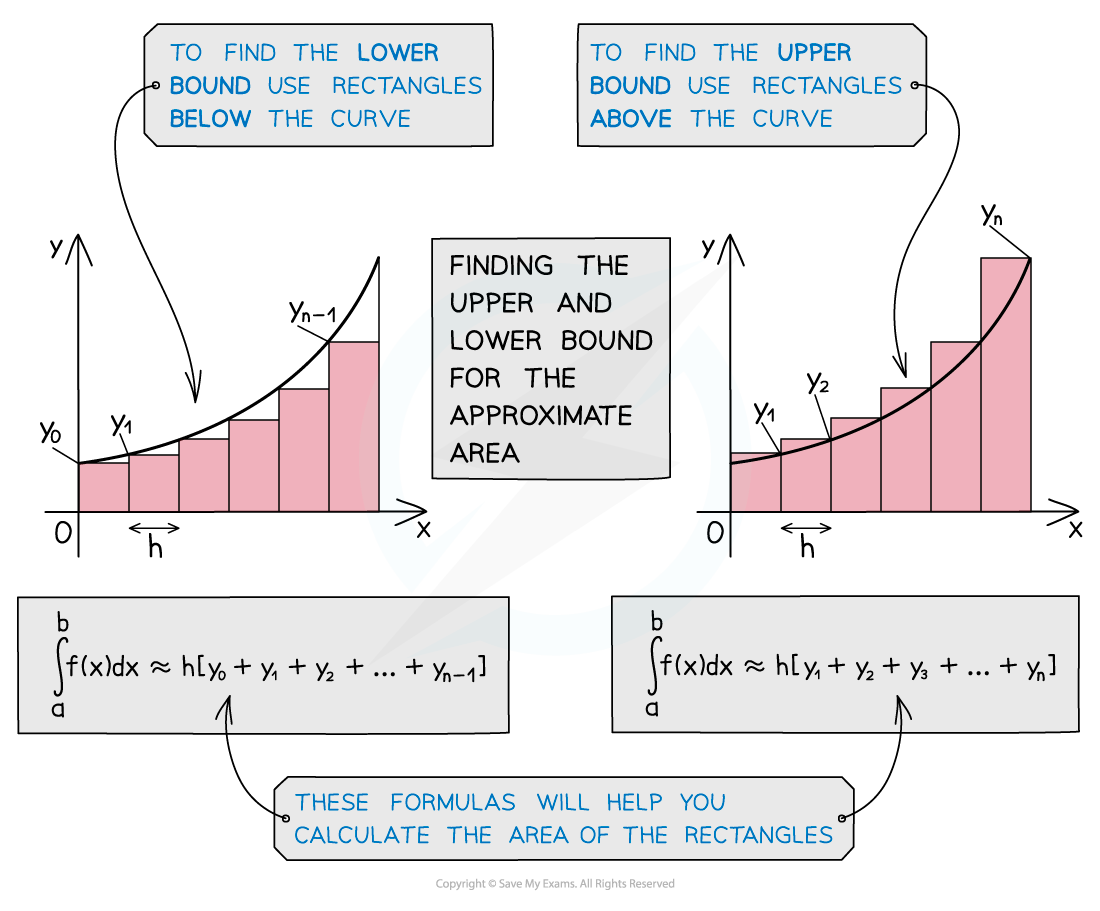#### Exam Tip

The formula for the trapezium rule is given in the formula booklet.

#### Worked Example